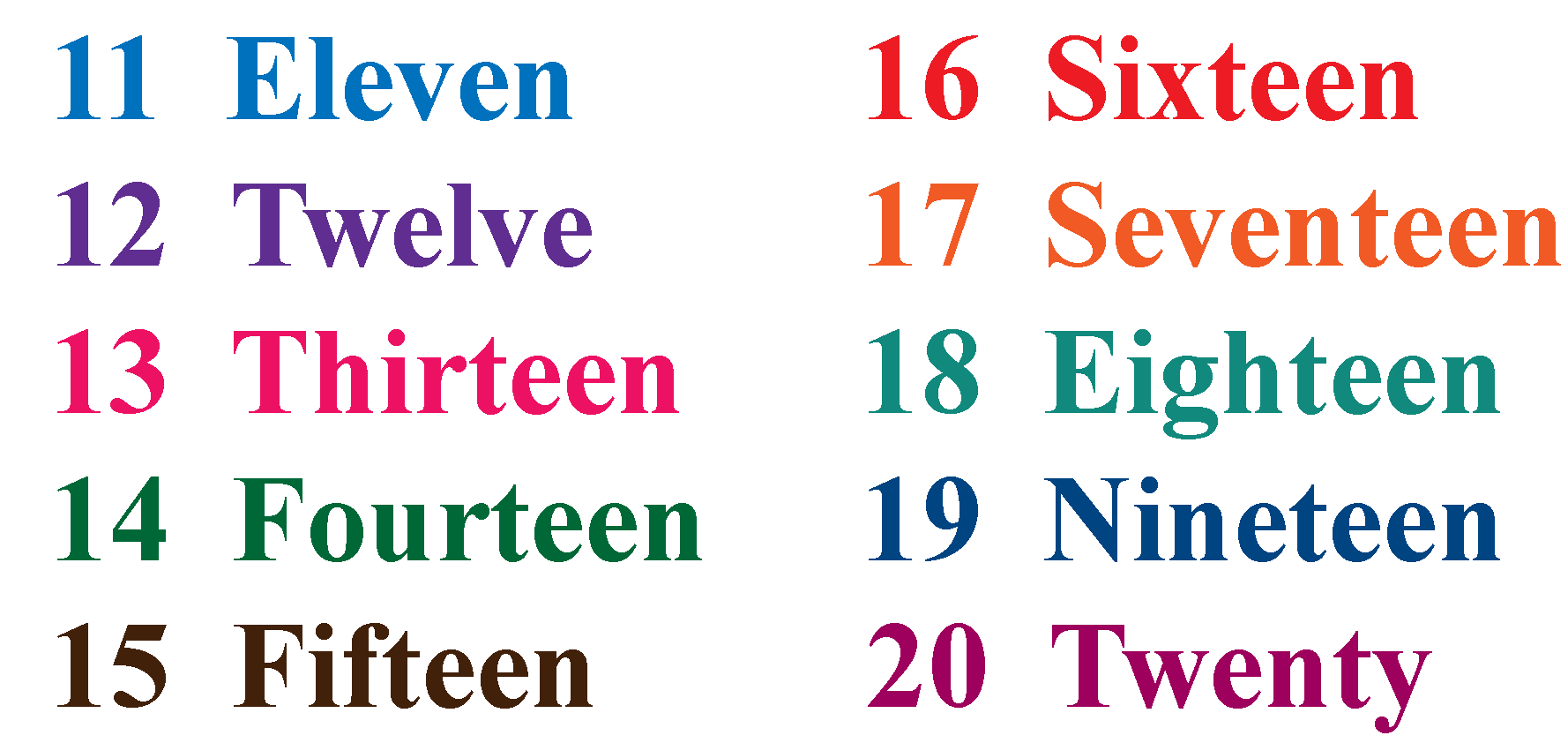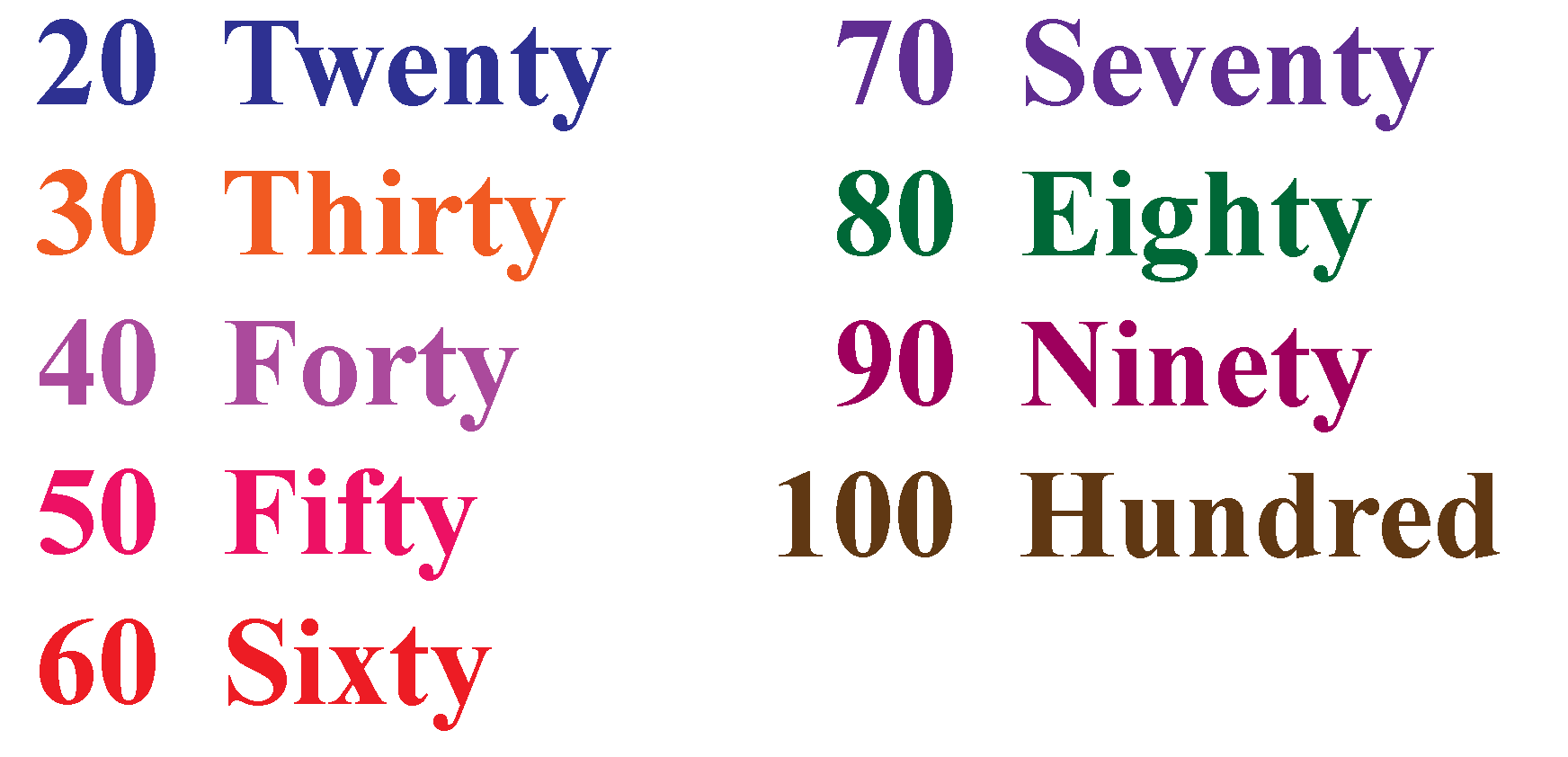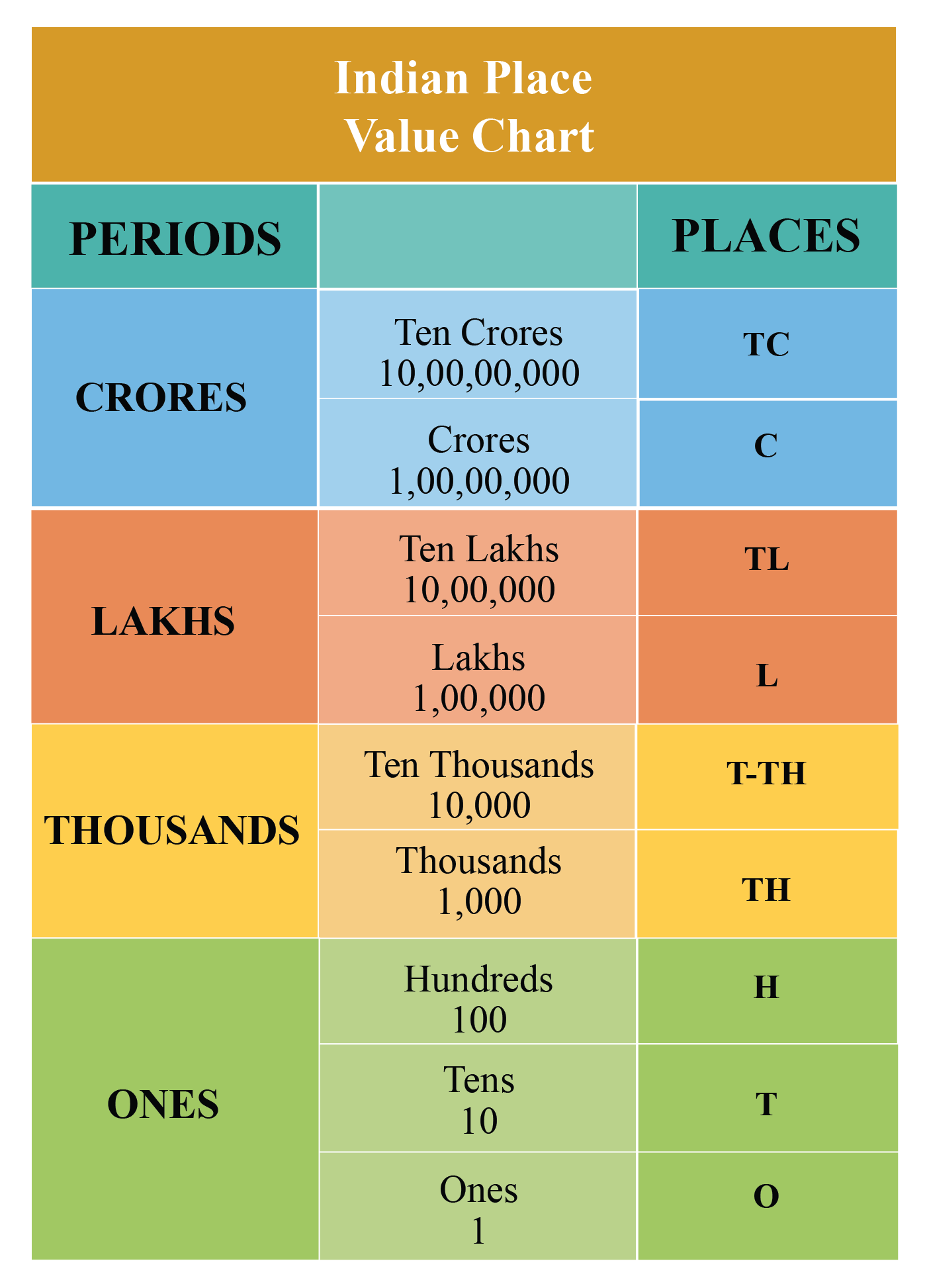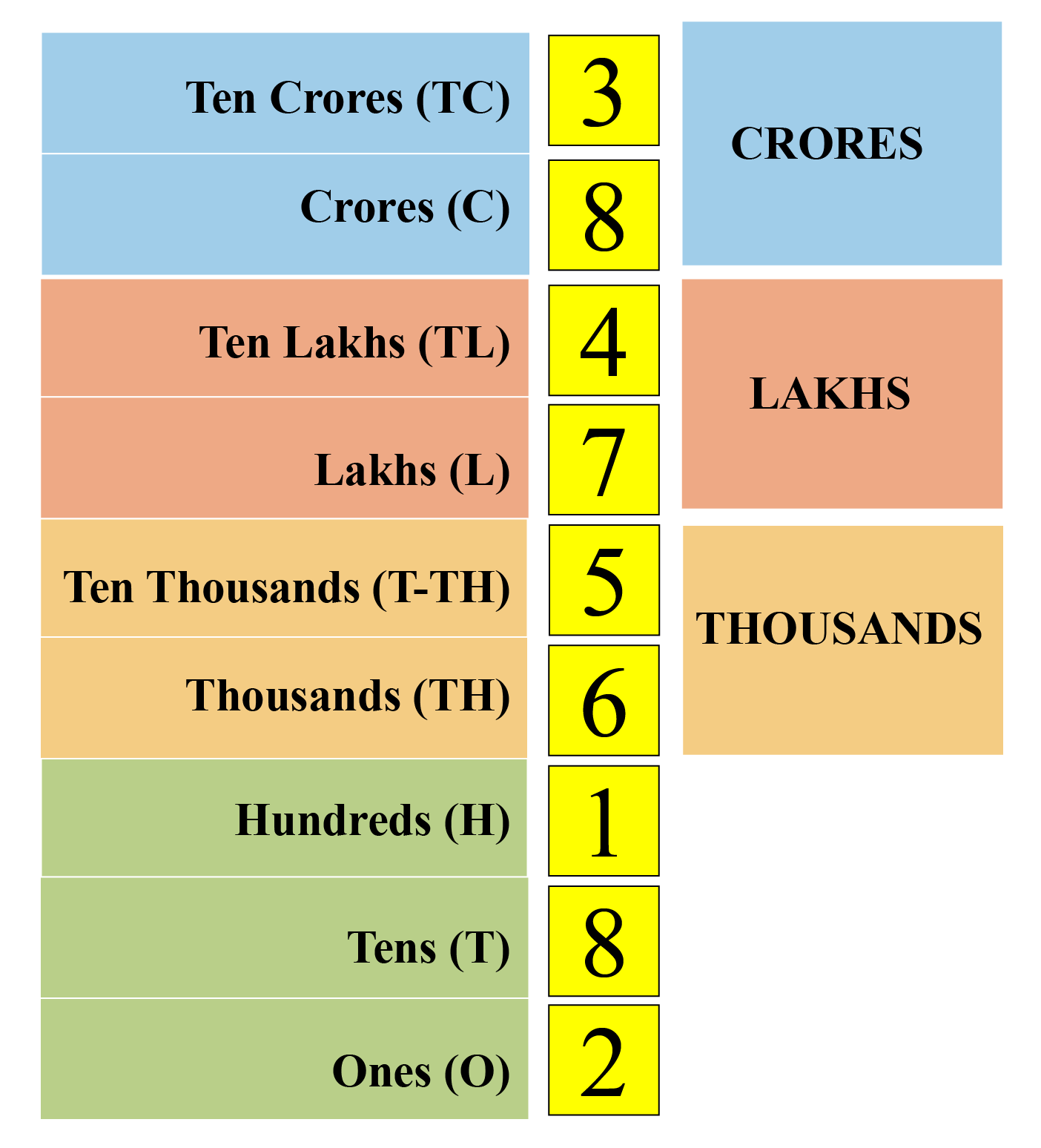# Numbers in Words

Numbers in Words
Go back to  'Numbers'

## Table of Contents

 1 Introduction to Numbers 2 Numbers In Words 3 How to Write Numbers in Words? 4 Important Notes on Numbers in Words 5 Conversion of Numbers in Words 6 Tips and Tricks 7 Practice Questions on Numbers in Words 8 Maths Olympiad Sample Papers 9 Frequently Asked Questions (FAQs)

We at Cuemath believe that Math is a life skill. Our Math Experts focus on the “Why” behind the “What.” Students can explore from a huge range of interactive worksheets, visuals, simulations, practice tests, and more to understand a concept in depth.

Book a FREE trial class today! and experience Cuemath's LIVE Online Class with your child.

## Introduction to Numbers

Long before numbers were invented, a unique mark was made on each item being counted.

This was a complex system for writing large numbers.

People realized that there was a need to adopt a standard system of counting.

This is how numbers came into existence.

In this Cuemath video, you will learn the primary components in Base Ten Blocks which is a hands-on way to understand number concepts.

## Numbers In Words

### Counting Numbers in Words

Numbers in words refer to the numbers written in alphabetical form.

A specific word is used to refer to each number.

For example, look at the following list of numerals and words accompanying each one of them.

You can observe in the above example that the numbers from 1 to 10 are written using specific words.

The words for the numbers from 11 to 20 are different from the words for other numbers.Now that you have learnt counting numbers in words from 1 to 20, let's learn the words for the numbers 30, 40, 50, 60, 70, 80, 90 and 100.Using these words, you can learn the words for other larger numbers.

## How to Write Numbers in Words?

To write a number in words in English, you should know the place value of each digit in the number.

The Indian place value chart is used to find the value of each digit in a number based on its position.

In order to read and write numbers, we use the following Place Value Chart.In this chart, the nine places are grouped into four periods: Ones, Thousands, Lakhs and Crores.

Starting from the right, the first period is ones, consisting of three place values: ones, tens and hundreds.

The second period is thousands, consisting of two place values: thousands and ten thousands.

The third period is lakhs, consisting of two place values: lakhs and ten lakhs.

The fourth period is crores, consisting of two place values: crores and ten crores.

While reading a number, all the digits in the same period are read together along with the name of the period, except the ones period.

Let's try out reading a large number.

Jagat owns a huge farm.

He sold his entire wheat harvest for Rs. 384756182.Let us try to read this big number, "384756182", using the Indian numeral system.

First, we will place it in the Indian place value chart.

Starting from the right-most number, this number represents the ones digit.It is easy to read any large number if we place the number in the place value chart of the Indian numeral system.

Therefore, the number is "Thirty eight crore forty seven lakh fifty six thousand one hundred and eighty two".

More Important Topics
Numbers
Algebra
Geometry
Measurement
Money
Data
Trigonometry
Calculus